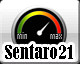# 3d graph code

2 replies to this topic

### #1PhantomOverrideAlpha

PhantomOverrideAlpha

Casio Fan

•• Members
•• 44 posts
• Gender:Male
• Location:Massillon Ohio USA
• Interests:Coding
Hacking
music
porting

• Calculators:
casio FX-9750GII
casio FX-9860GIII
Casio FX-9750GIII

Posted 25 December 2018 - 11:34 PM

I have a code that I wrote that I want people to try
Here is the code
Format:MCS1
Type Number:1
File Name:GRAPH D3
Option Name:
Communication SW:0
Capacity:2602

1 ->S
BG-None
Lbl 0
0 ->E
{ (-)9,9,18,0.4, (-)9,9,18,1} -> List 20
1 -> Dim List 17
" cos sqrt(X x^2+Y x^2)" -> Y 1
Lbl 1
0 ->W
ClrGraph
ViewWindow 1,127,0,1,63,0
F-Line 5,10,5,63
F-Line 1,57,127,57
F-Line 124,10,124,63
Text 1,10,"3D GRAPH 1/1"
Text 10,10,"F1 : EQUATION"
Text 17,10,"F2 : SAUVER IMAGE"
Text 24,10,"F3 : TABLEAU"
Text 57,1,"Z= IMG TAB"
Text 38,10,"Z="
1 ->X
5 ->Y
If E=1
Then Text 38,19,"UNKNOW "
Y 1= cos sqrt(X x^2+Y x^2) => Text 38,19," cos sqrt(X x^2+Y x^2)"
Y 1= cos X+ sin Y => Text 38,19," cos X+ sin Y"
Y 1=X x^2+Y x^2 => Text 38,19,"X x^2+Y x^2"
Y 1=Y x^2-X x^2 => Text 38,19,"Y x^2-X x^2"
Y 1=X x^2-Y x^2 => Text 38,19,"X x^2-Y x^2"
Y 1= sqrt(Y x^2+X x^2) => Text 38,19," sqrt(Y x^2+X x^2)"
IfEnd
StoPict 18
Do
Do
Getkey
LpWhile Not Ans
Ans=79 => Goto2
Ans=69 => Goto5
Ans=59 => Goto6
If Ans=78
Then Do
Do
Getkey
LpWhile Not Ans
Ans=58 => Goto3
If Ans=47
Then ClrList20
ClrMat A
ClrMat B
"" -> Y 1
Stop
IfEnd
LpWhile 1=1
IfEnd
If Ans=68
Then Do
0 ->W
Do
Getkey
LpWhile Not Ans
Ans=59 => GotoS
LpWhile 1=1
IfEnd
LpWhile Ans <>31
Goto4
Lbl 2
ClrText
"3D GRAPH EQUATION : Z1= cos ( sqrt(X x^2+Y x^2)) Z2= cos (X)+ sin (Y) Z3=X x^2+Y x^2 Z4=<?>"
1 ->A
Do
Locate 1,1+A," ->"
Do
Getkey
LpWhile Not Ans
Locate 1,A+1," "
Ans=47 => Goto1
Ans=28 =>A-1 ->A
Ans=37 =>A+1 ->A
LpWhile Ans <>31
1 ->E
A=1 =>" cos sqrt(X x^2+Y x^2)" -> Y 1
A=2 =>" cos X+ sin Y" -> Y 1
A=3 =>"X x^2+Y x^2" -> Y 1
If A=4
Then "EQUATION":? -> Y 1
IfEnd
Goto1
Lbl 3
ClrText
"3D ViewWindow : Xmin: Ymin: Xmax: Ymax: XGRD: YGRD: ZFCT: ZINC:"
For 0 ->A To 1
For 1 ->B To 4
Locate 6+11A,B+1, List 20[4A+B]
Next
Next
Locate 2,6," : CHANGER"
Locate 2,7," : OK"
Do
Getkey
LpWhile Ans <>31 And Ans <>44
If Ans=44
Then ClrText
"ENTER NEW VALUES:"
" Xmin":? -> List 20
" Xmax":? -> List 20
"XGRID":? -> List 20
" Ymin":? -> List 20
" Ymax":? -> List 20
"YGRID":? -> List 20
"Z Factor (ZOOM)":? -> List 20
"ZINC(OFFSET)":? -> List 20
Goto3
IfEnd
Goto1
Lbl 4
ClrGraph
List 20 ->M
List 20 ->N
( Xmax- Xmin-2)/M ->U
( Ymax- Ymin-1)/N ->V
2/M ->W
{M,N -> Dim Mat A
( List 20- List 20)/M ->P
( List 20- List 20)/N ->Q
ClrText
Locate 1,1,"CHARGEMENT..."
Locate 6,4,"POURCENTS"
For 1 ->A To M
Locate 3,4, Int (100((A-1)/M
For 1 ->B To N
AP+ List 20 ->X
BQ+ List 20 ->Y
( Y 1+ List 20)* List 20 -> Mat A[A,B
Next
Next
Lbl D
For 1 ->A To M-1
For 1 ->B To N-1
Xmin+1.5+AU-BW ->G
Ymin+BV ->H
Mat A[A,B] ->I
Mat A[A,B+1] ->J
Mat A[A+1,B] ->K
F-Line G,H+I,G-W,H+V+J
F-Line G,H+1,G+U,H+K
Next
Xmin+1.5+AU-NW ->G
Ymin+NV ->H
F-Line G,H+ Mat A[A,N],G+U,H+ Mat A[A+1,N]
Next
For 1 ->B To N-1
Xmin+1.5+MU-BW ->G
Ymin+BV ->H
F-Line G,H+ Mat A[M,B],G-W,H+V+ Mat A[M,B+1]
Next
StoPict 18
0 ->K
Do
Getkey ->K
If K=79
Then ClrText
"ZOOM Factor "
? ->I
I Mat A -> Mat A
ClrGraph
GotoD
IfEnd
LpWhile K <>47
Goto1
Lbl 5
Prog "IMG"
Goto1
Lbl 6
0 ->T
0 ->V
5 ->U
5 ->W
Lbl 7
{(T+U+1)*(V+W+1),3} -> Dim Mat B
1 ->C
ClrText
Locate 1,1,"CHARGEMENT..."
Locate 7,4,"POURCENTS"
For T ->X To U
Locate 3,4, Int (100*(X+1)*(Y+1)/((T+U+1)*(V+W+1)))
For V ->Y To W
Y 1 -> Mat B[C,3
Y -> Mat B[C,2
X -> Mat B[C,1
1+C ->C
Next
Next
Mat B Disp Do
If Getkey=58
Then ClrText
Do
Locate 1,1,"REGLAGE TABLEAU"
Locate 2,3," Xmin="
Locate 8,3,T
Locate 2,4," Xmax="
Locate 8,4,U
Locate 12,3," Ymin="
Locate 18,3,V
Locate 12,4," Ymax="
Locate 18,4,W
LpWhile Getkey <>31
IfEnd
LpWhile Getkey <>47
Goto1
ClrMat
ClrList
"" -> Y 1
Goto0
Lbl S
ClrText
Locate 1,1," PARAMETRES :"
Locate 3,3,"ANGLES :"
S=2 => Locate 12,3," Deg ( Deg>)"
Locate 4,5," : OK"
Locate 4,6," : CHANGER"
Do
Getkey
LpWhile Ans <>44 And Ans <>31
If Ans=44
Then ClrText
Locate 3,1,"UNITE ANGULAIRE :"
Locate 4,4,"DEGRES ( Deg>)"
2 ->X
Do
Locate 2,X+1," ->"
Do
Getkey
LpWhile Not Ans
Locate 2,X+1," "
Ans <>31 =>X+( Ans-33.5)/4.5 ->X
LpWhile Ans <>31
X=3 => Deg
X-1 ->S
GotoS
IfEnd
Goto0

### #2PhantomOverrideAlpha

PhantomOverrideAlpha

Casio Fan

•• Members
•• 44 posts
• Gender:Male
• Location:Massillon Ohio USA
• Interests:Coding
Hacking
music
porting

• Calculators:
casio FX-9750GII
casio FX-9860GIII
Casio FX-9750GIII

Posted 28 December 2018 - 08:55 PM

### #3sentaro21

sentaro21

Casio Technician

•• Members
•• 361 posts
• Gender:Male
• Location:JAPAN

• Calculators:
FX-603P fx-4800P fx-5800P
CFX-9850GC PLUS
fx-9860G
fx-9860GII
fx-9860GII-2
fx-9860GII-2 SD
fx-CG10
fx-CG20
fx-CG50
HP-Prime
HP 50G
TI-Nspire CX CAS
TI-84+CE

Posted 29 December 2018 - 11:44 AM

Prog "IMG" seems to be missing, but it is works well.